top of page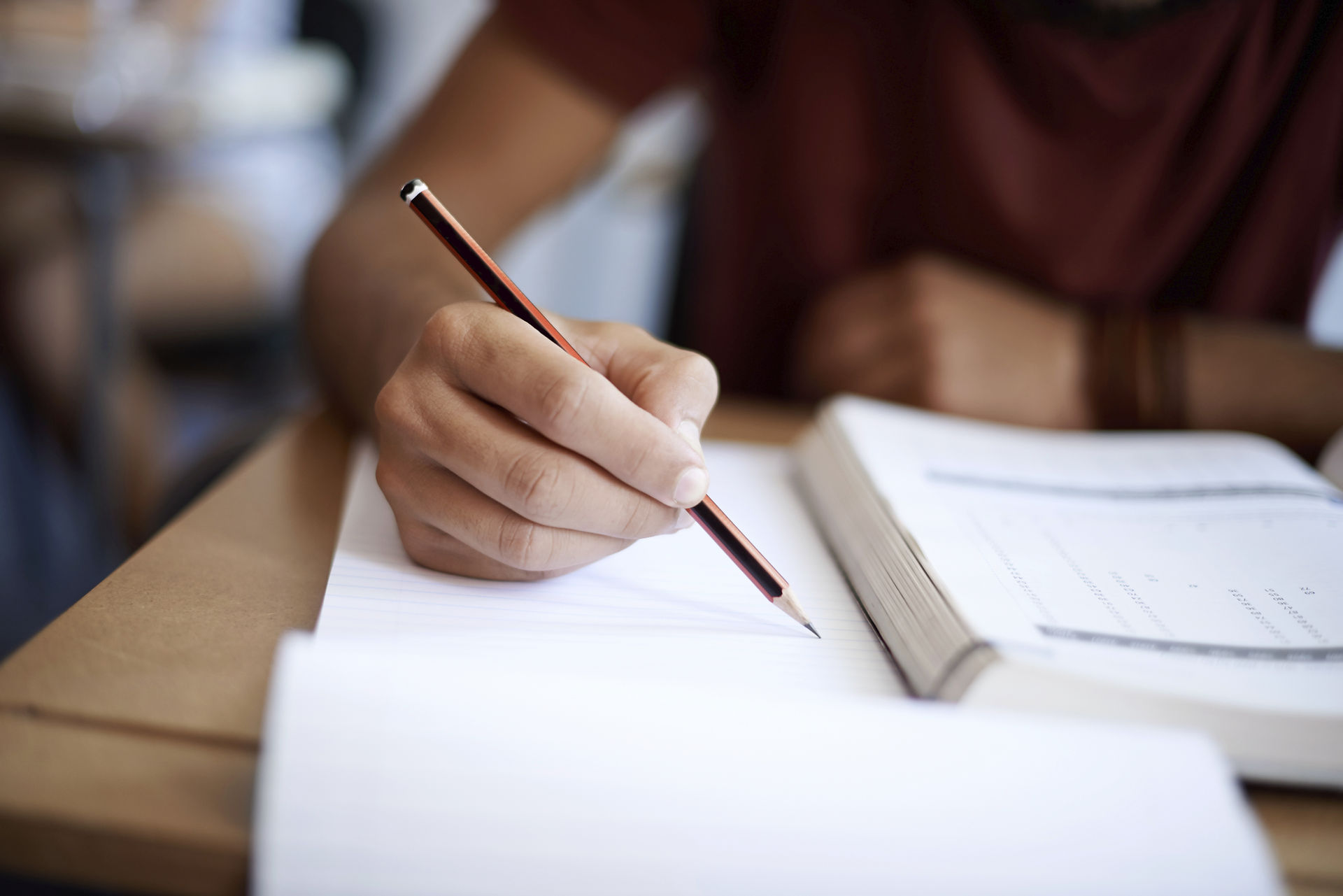• # GATE Previous Year Questions

Matrix:

Collection of numbers arranged in rows and columns. An 'm x n' matrix has m rows and n columns.

A 2 x 3 matrix having 2 rows (arranged horizontally) and 3 columns (arranged vertically) is represented as:

Definition​Matrix as linear transformation of vectors:

A matrix may be represented as linear transformation of vectors in space. Consider a 2x2 matrix

​Matrix as linear transformation of vectors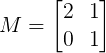This is a linear transformation matrix for a vector in 2-D plane. If 'i' and 'j' are unit vectors along x-axis and y-axis respectively, then column 1 of the above matrix represents position vector of transformed 'i' and column 2 represents position vector of transformed 'j'.i (transformed) = 2*i + 0*j = 2i

j (transformed) = 1*i + 1*j = i+j

For example a vector V = 2i + 3j is transformed by Matrix M as follows: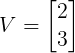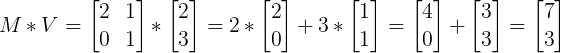Example:

GATE 2019: The transformation matrix for mirroring a point in x-y plane about the line y = x is given by

GATE Questions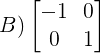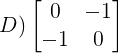Solution:

Transform matrix is such that, all transformed vectors in x-y plane are mirror image of original vector. First task is to find out transformed unit vectors 'i' and 'j'.Mirror image of 'i' about line y = x is 'j' and mirror image of 'j' about same is 'i'.

Hence,

i (transformed) = j = 0*i + 1*j

j (transformed) = i = 1*i + 0*j

In matrix form above transformation may be written as: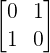Hence the correct answer is 'C'.

Example:

GATE 2014: Which one of the following equation is correct identity for arbitrary 3x3 real matrix P, Q and R.

A) P(Q + R) = PQ + RP

B) (P - Q)^2 = P^2 - 2PQ + Q^2

C) Det (P + Q) = Det P + Det Q

D) (P + Q)^2 = P^2 + PQ + QP + Q^2

Solution:

P(Q + R) = PQ + PR

(P-Q)^2 = P^2 - PQ - QP + Q^2

Det (P + Q) ≠ Det P + Det Q

(P+Q)^2 = P^2 + PQ + QP + Q^2

Hence option D is the correct answer.

bottom of page